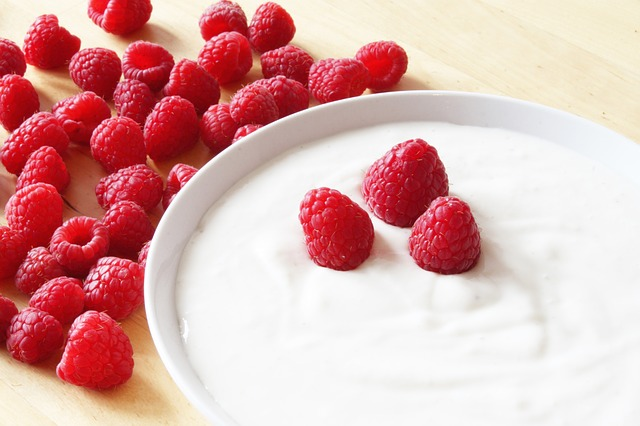## 13.6 Compiling tables of numerical summary information

Here are some tips for compiling tables of numerical summary information:

• Round numbers appropriately (don’t necessarily use all decimals provided by software).
• Place captions above tables.
• In general, use no vertical lines and very few horizontal lines.
• Align numbers in the table by decimal point when possible, for easier reading.
• Ensure the table allows readers to easily make the important comparisons.Example 13.12 (Tables for summarising data) Consider a study assessing the effects of probiotic and conventional yoghurt on blood glucose and antioxidant status in Type 2 diabetic patients. A randomised controlled trial (i.e., an experiment) collected data from 60 patients.

Compare the two numerical summary tables in Tables 13.6 and 13.7: Table 13.6 makes comparing the two groups easier, but Table 13.7 is the more conventional orientation (for practical purposes: fewer columns).
TABLE 13.6: Baseline characteristics of study participants. A superscript a indicates the data are summarised using means $$\pm$$ standard deviation; a superscript b indicates the data are summarised using medians $$\pm$$ IQR
Yoghurt Age$$a$$ Weight (kg)$$a$$ BMI$$a$$ (kg/m$$2$$) Metformin/d$$b$$ Glibenclamide/d$$b$$
Conventional ($$n=30$$) $$51.0 \pm 7.3$$ $$75.42 \pm 11.28$$ $$29.14 \pm 4.30$$ $$2 \pm 1.25$$ $$1 \pm 1$$
Probiotic ($$n=30$$) $$50.9 \pm 7.7$$ $$76.18 \pm 10.94$$ $$28.95 \pm 3.65$$ $$2 \pm 1.25$$ $$2 \pm 2$$
TABLE 13.7: Baseline characteristics of study participants. A superscript a indicates the data are summarised using means $$\pm$$ standard deviation; a superscript b indicates the data are summarised using medians $$\pm$$ IQR
Variable Conventional yoghurt ($$n=30$$) Probiotic yoghurt ($$n=30$$)
Age$$a$$ 51.00 $$\pm$$ 7.32 50.87 $$\pm$$ 7.68
Weight (kg)$$a$$ 75.42 $$\pm$$ 11.28 76.18 $$\pm$$ 10.94
BMI (kg/m2)$$a$$ 29.14 $$\pm$$ 4.30 28.95 $$\pm$$ 3.65
Metformin/d$$b$$ 2 $$\pm$$ 1.25 2 $$\pm$$ 1.25
Glibenclamide/d$$b$$ 1$$\pm$$ 1 2 $$\pm$$ 2
Think 13.11 (Sample differences) Do you think a difference exists between the mean BMI in the two groups in the population, based on Tables 13.6 and 13.7? Explain.

### References

Ejtahed HS, Mohtadi-Nia J, Homayouni-Rad A, Niafar M, Asghari-Jafarabadi M, Mofid V. Probiotic yogurt improves antioxidant status in type 2 diabetic patients. Nutrition. 2012;28:539–43.